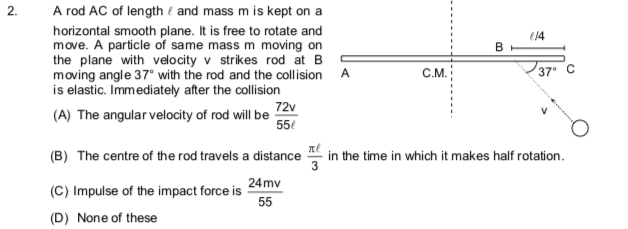# Doubt from RotationFor 3 . Draw FBD . Use time period =2pir/v. Find r in terms of h and find v by centripetal force

1 Like

yes I got it bro..thanks

not getting this.. @Achyut_2020 ..the 1st problem

I'm getting ABC

correct..sol pls

You have to solve these equations . Use this to find w and velocity of com in terms of v . Now for impulse calculate change in momentum and for distance travelled = velocity of com × time , where time = pi/w

1 Like

Got now